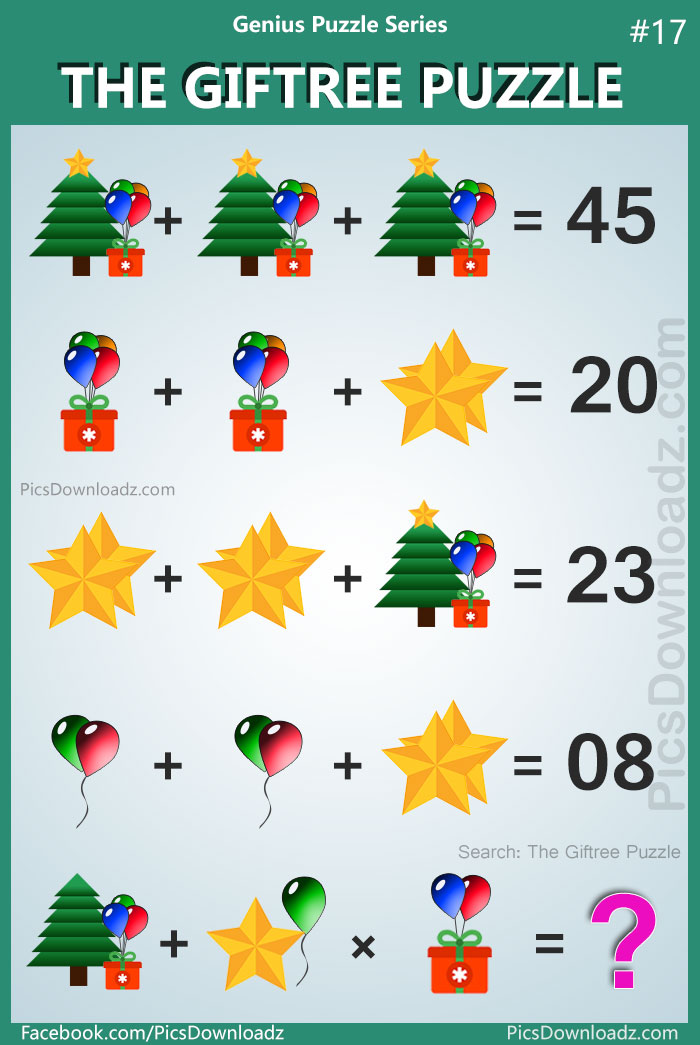This post may contain affiliate links. This means if you click on the link and purchase the item, We will receive an affiliate commission at no extra cost to you. See Our Affiliate Policy for more info.

0
33744

### Confusing logical math puzzles; 95% will fail to attempt this logic math puzzle. “The Giftree Puzzle” Difficult Math Puzzles with answer!

Welcome back to the genius puzzle series. Another awesome puzzle for you guys “The Giftree (Gift+Tree) Puzzle”.  The Items used in this puzzle names as follow: Tree, Gift, Star, Balloons

If you already go through my previous puzzle “The Tree Puzzle” then this logical puzzle is going to be very simple for you. Lookout for the details, apply the logic and find out the true values of each item to solve the puzzle.

Let see, how many of you can solve this viral puzzle. Check out the image and share your equation or answer in the comment section below. Best of luck!

### The Giftree Puzzle: Genius Puzzle Series #17### Want to print or share on your blog?

Simply download this puzzle image and print it out. Share this puzzle with your kids, students, and fellows. And for blog and website, you are free to use this image but you’re not allowed to edit the image and must attribute the image by linking to the original source, so we will be able to continue creating new puzzles & other amazing posts for you. Love <3

## Solution:

### Equation 1:

⇒ 15 + 15 + 15 = 45

### Equation 2:

⇒ 8 + 8 + (2+2) = 20 (One Star = 2 from equation 3)

### Equation 3:

⇒ (2+2) + (2+2) + 15 = 23

⇒ One Star = 2

### Equation 4:

⇒ (1+1) + (1+1) + (2+2) = 8

⇒ One Balloon = 1

### Equation 5:

Here are some key points before going to solve this equation

• Tree (5 Layers Vs 6 Layers), Tree (with Star Vs without Star)
• Gift (with Balloon Vs without Balloon)

Last Equation written as follow:

⇒ [Tree(6 layers, without star)+ Gift (with 3 balloons)] + [One Star + One Balloon] + [Gift (with 2 Balloons)]

As we know now: Single Star= 2, Single Balloon = 1

Gift (with 4 balloons) = 8 ( from equation 2nd)

Therefore Gift (without Balloons) = 8 – 4 = 4

Now, if we calculate the value of Three from the 1st equation we get;

Tree + Star + Gift(with balloons) = 15

Tree + 2 + 8 = 15  ⇒  Tree + 10 = 15  ⇒  Tree = 15 – 10 = 5

Value of Tree = 5 (in first equation)

But here is the twist: In the 1st equation, The Tree has only 5 layers but in the last equation the Tree has 6 layers.

What is the logic? Tree(5 layers) = 5  Vs Tree(6 layers) = 6

### Now rewrite the last equation:

⇒ [Tree(6 layers, without star)+ Gift (with 3 balloons)] + [One Star + One Balloon] + [Gift (with 2 Balloons)]

(Tree = 6 as it has 6 layers ; Star = 2; Balloon = 1 ; Gift (without) = 4)

⇒ (6+4+3) + (2 + 1) × 4+2

⇒ (13) + (3) × (6)

⇒ 13 + 18 = 31 Answer

Got it? these logical puzzles are interesting, isn’t it? Enjoy 😉

Search items:

The Giftree Puzzle, Genius Puzzle Series, Puzzle 17, Viral Math Puzzles, Hard Math Puzzles Images, Facebook Math Puzzle Image, Viral Whatsapp Image Puzzle, Tree Star Gift Balloon Puzzle, Tree and Gift Puzzles, Puzzles for Adults Logical Puzzle Question, Genius Puzzles Images, Only for Genius Puzzles, Genius Puzzle Series 17, Viral Facebook Puzzle, Viral Whatsapp Puzzle, The Gift Tree Puzzle, Facebook Tree Puzzle.